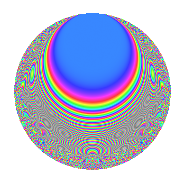# Properties

 Label 27.4.eLevel 27 Weight 4 Character orbit e Rep. character $$\chi_{27}(4,\cdot)$$ Character field $$\Q(\zeta_{9})$$ Dimension 48 Newform subspaces 1 Sturm bound 12 Trace bound 0

# Related objects

## Defining parameters

 Level: $$N$$ = $$27 = 3^{3}$$ Weight: $$k$$ = $$4$$ Character orbit: $$[\chi]$$ = 27.e (of order $$9$$ and degree $$6$$) Character conductor: $$\operatorname{cond}(\chi)$$ = $$27$$ Character field: $$\Q(\zeta_{9})$$ Newform subspaces: $$1$$ Sturm bound: $$12$$ Trace bound: $$0$$

## Dimensions

The following table gives the dimensions of various subspaces of $$M_{4}(27, [\chi])$$.

Total New Old
Modular forms 60 60 0
Cusp forms 48 48 0
Eisenstein series 12 12 0

## Trace form

 $$48q - 6q^{2} - 6q^{3} - 6q^{4} + 6q^{5} - 18q^{6} - 6q^{7} - 75q^{8} - 54q^{9} + O(q^{10})$$ $$48q - 6q^{2} - 6q^{3} - 6q^{4} + 6q^{5} - 18q^{6} - 6q^{7} - 75q^{8} - 54q^{9} - 3q^{10} + 57q^{11} + 147q^{12} - 6q^{13} + 51q^{14} - 36q^{15} + 18q^{16} - 207q^{17} - 639q^{18} - 3q^{19} - 597q^{20} - 138q^{21} - 60q^{22} + 402q^{23} + 1170q^{24} - 222q^{25} + 1914q^{26} + 1125q^{27} - 12q^{28} + 480q^{29} + 459q^{30} - 60q^{31} - 648q^{32} - 639q^{33} + 288q^{34} - 1257q^{35} - 2088q^{36} - 3q^{37} - 1524q^{38} - 768q^{39} + 561q^{40} - 1731q^{41} - 3078q^{42} + 507q^{43} - 2211q^{44} - 360q^{45} - 3q^{46} + 984q^{47} + 2289q^{48} - 600q^{49} + 4359q^{50} + 2655q^{51} - 1431q^{52} + 2736q^{53} + 5454q^{54} - 12q^{55} + 5907q^{56} + 3426q^{57} - 897q^{58} + 2238q^{59} + 1314q^{60} + 48q^{61} - 2118q^{62} - 2610q^{63} - 195q^{64} - 6990q^{65} - 11115q^{66} - 681q^{67} - 11169q^{68} - 6138q^{69} - 33q^{70} - 3105q^{71} - 36q^{72} - 219q^{73} + 3543q^{74} + 2604q^{75} + 3426q^{76} + 4722q^{77} + 6066q^{78} + 2802q^{79} + 9870q^{80} + 3438q^{81} - 12q^{82} + 3468q^{83} + 7674q^{84} + 2529q^{85} + 3624q^{86} + 2880q^{87} + 2850q^{88} - 5202q^{89} - 12510q^{90} + 267q^{91} - 18453q^{92} - 11802q^{93} - 1653q^{94} - 10113q^{95} - 14094q^{96} - 3381q^{97} - 4392q^{98} - 1242q^{99} + O(q^{100})$$

## Decomposition of $$S_{4}^{\mathrm{new}}(27, [\chi])$$ into newform subspaces

Label Dim. $$A$$ Field CM Traces $q$-expansion
$$a_2$$ $$a_3$$ $$a_5$$ $$a_7$$
27.4.e.a $$48$$ $$1.593$$ None $$-6$$ $$-6$$ $$6$$ $$-6$$

## Hecke Characteristic Polynomials

There are no characteristic polynomials of Hecke operators in the database